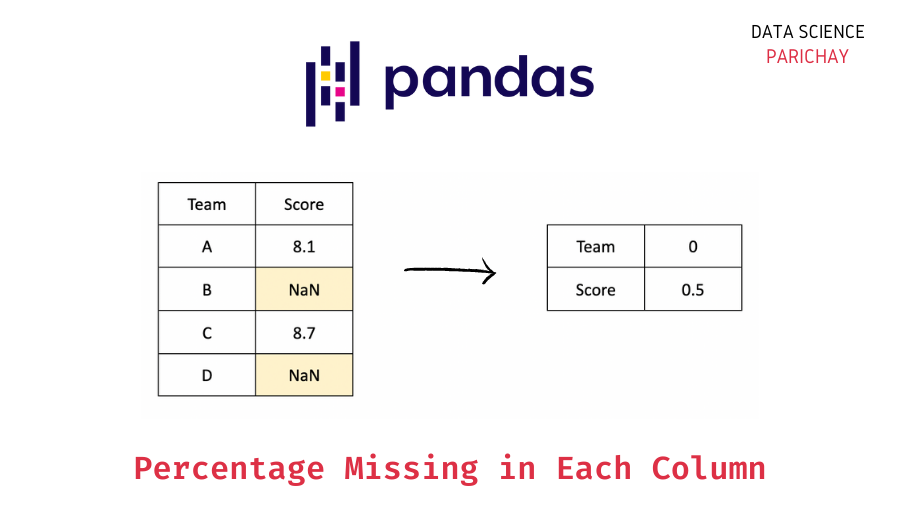# Pandas – Percentage of Missing Values in Each Column

In this tutorial, we will look at how to calculate the percentage of missing values in each column of a pandas dataframe with the help of some examples.

Let’s create a pandas dataframe that we will be using throughout this tutorial.

```import pandas as pd
import numpy as np

# create a dataframe of car models by two companies
df = pd.DataFrame({
'Name': ['Rob', 'Rob', 'Rob', 'Emma', 'Emma', 'Emma', 'Hasan', 'Hasan', 'Hasan'],
'Subject': ['English', 'Science', np.nan, 'English', 'Science', 'Maths', 'English', 'Science', 'Maths'],
'Marks': [67, 81, 59, np.nan, np.nan, 82, 73, 76, 54],
'Projects': [0, 1, 0, np.nan, 1, 0, np.nan, np.nan, np.nan]
})
# display the dataframe
df```

Output:

```    Name  Subject  Marks  Projects
0    Rob  English   67.0       0.0
1    Rob  Science   81.0       1.0
2    Rob      NaN   59.0       0.0
3   Emma  English    NaN       NaN
4   Emma  Science    NaN       1.0
5   Emma    Maths   82.0       0.0
6  Hasan  English   73.0       NaN
7  Hasan  Science   76.0       NaN
8  Hasan    Maths   54.0       NaN```

We now have a dataframe containing scores of some students in different subjects. Note that some values in the dataframe are missing.

## How to compute total missing values in a column?

For a pandas column, you can use a combination of the `isnull()` and the `sum()` function to compute the total number of missing values in a column.

For example, let’s compute the number of missing values in the “Projects” column.

```# total missing values in "Projects" column
df["Projects"].isnull().sum()```

Output:

`4`

We get the output as 4. You can see from the dataframe displayed above that this is correct.

📚 Data Science Programs By Skill Level

Introductory

Intermediate ⭐⭐⭐

🔎 Find Data Science Programs 👨‍💻 111,889 already enrolled

Disclaimer: Data Science Parichay is reader supported. When you purchase a course through a link on this site, we may earn a small commission at no additional cost to you. Earned commissions help support this website and its team of writers.

## Percentage of missing values in a column

You can similarly compute the percentage of missing values in a pandas dataframe column. Divide the total missing values with the length of the column to get the fraction of values missing in the column.

Let’s compute this for the same “Projects” column.

```# percentage missing values in "Projects" column
df["Projects"].isnull().sum()/len(df["Projects"])```

Output:

`0.4444444444444444`

We find that 44.44% of the values in the column “Price” are missing.

## Percentage of missing values in each column

You can similarly extend the above step to each column in the dataframe. Instead of applying the `isnull()` function to a single column, apply it to the entire dataframe. Let’s see it in action.

```# percentage missing values in the dataframe
df.isnull().sum()/len(df)```

Output:

```Name        0.000000
Subject     0.111111
Marks       0.222222
Projects    0.444444
dtype: float64```

Here, we get the proportion of missing values in each column of the dataframe `df`. You can see that the column “Name” column does not have any missing values, the “Subject”, “Marks”, and the “Projects” columns have 11.11%, 22.22%, and 44.44% values missing respectively.

You might also be interested in –

•